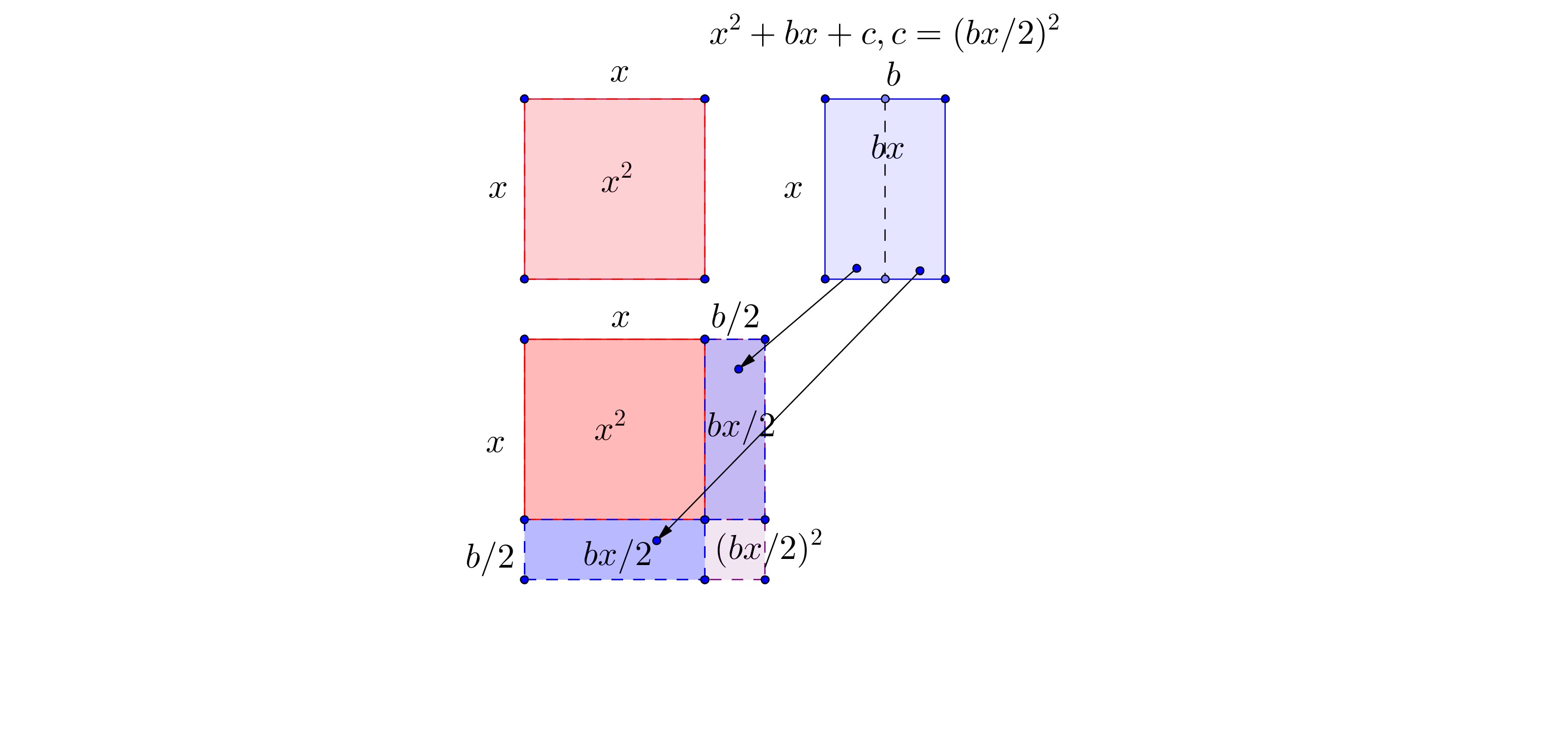# POLQ 2 | Lesson 2 | Watch (Completing the Square)The following illustration allows us to look at the process of completing the square both algebraically and pictorially. Below, is the generic expression in the form of $$x^2 +bx$$. Created using Geogebra.Steps to Completing the Square with tiles:

1) The x^2 is represented by a square.

2) The rectangle next to it has the area of bx as its length is x and its width is b.

3) Divide the rectangle bx in half. One of the two resulting rectangles increases the length of the sides of the square.

4) Determine the number of units missing to complete the square.  This number can be seen visually but also found by dividing by 2 and squaring the quotient.  Add that number to the expression as part of the trinomial and subtract it outside of the trinomial so the value of the expression does not change.

5) The completed square is the length of the side of the square, squared and the number that was subtracted from the expression.

The goal is to get the expression in the form of $$(x+d)^2+e$$ where $$d$$ is the square root of $$c$$ in a perfect square trinomial and $$e$$ is the inverse of what was added to $$x^2 +bx$$ to complete the square. Note that in this example the value of $$c$$ is 0.

Here is an equation to use as an example :

$$x^2 + 2x +12 = 0$$

1. Divide all terms by the a term, if $$a ≠1$$.   $$x^2 +2x +12 = 0$$
2. Move the constant to the right side of the equal sign. $$x^2 +2x = -12$$
3. Divide the b in half and then square it to find c.  Add to both sides.                                        $$x^2 +2x +1 = -12+1$$
4. Simplify each side.$$(x+1)^2 =-11$$
5. Rearrange into the expression form  $$(x+d)^2 +e$$.  $$(x+1)^2 +11$$

Watch video for additional examples:

Completing the square (video) | Khan Academy

Worked example: Completing the square (intro) | Khan Academy